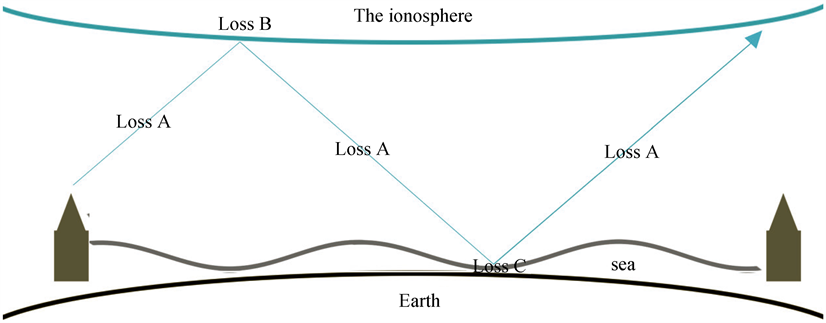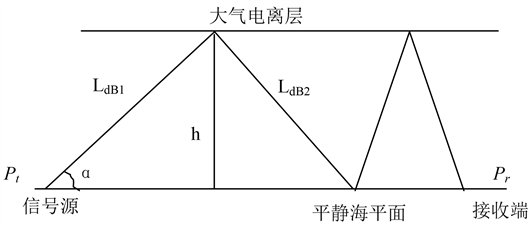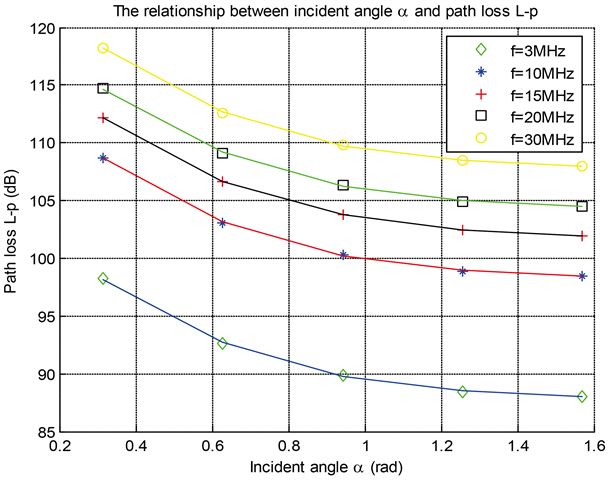﻿ 高频信号下海平面无线通信性能研究

# 高频信号下海平面无线通信性能研究Study on Performance of Sea Level Wireless Communication under High Frequency Signal

Abstract: This thesis mainly studies the multi hop propagation mode of high frequency radio electromagnetic wave, and establishes a mathematical model of the reflection of high frequency electromagnetic wave signals in ionosphere and sea surface. By analyzing the dynamic characteristics of sea water, the reflection process of the signal in calm sea and rough sea is simulated, and the transmission loss is quantitatively and qualitatively studied. On the premise of reasonable hypothesis, the calculation formula of maximum propagation hops in multi hop mode is obtained, the influence of related variables on jumping number is analyzed and the changed curve is drawn.

1. 引言

3~30 MHz的高频电磁波信号也称为短波或天波，主要依靠电离层对短波的一次或多次反射进行长距离无线电通信传输  ，可以在电离层和地面之间连续跳跃式前进，由于电离层是不稳定的，它的厚度、高度和密度随时都在变化，白天更加明显，因此电离层的反射面特性决定了反射强度和信号在保持有用信号完整性的情况下，最终能传输多远，MUF之上的频率穿过电离层进入太空   。

2. 多跳模式传播损耗模型

${L}_{dB}=m\cdot LossA+n\cdot LossB+v\cdot LossC$ (1)Figure 1. Multi hop transmission modeFigure 2. Multi hop propagation loss model of high frequency electromagnetic wave between ionosphere and sea level

$LossA={L}_{dB1}+{L}_{dB2}$

${L}_{dB}=2\cdot \left({L}_{dB1}+{L}_{dB2}\right)+LossB+LossC$

2.1. 高频电波在大气间传播损耗模型

$\frac{{P}_{t}}{{P}_{r}}=\frac{{\left(4\text{π}d\right)}^{2}}{{\lambda }^{2}}=\frac{{\left(4\text{π}fd\right)}^{2}}{{c}^{2}}$ (2)

${L}_{dB1}=10\mathrm{lg}\frac{{P}_{t}}{{P}_{r}}==20\mathrm{lg}\frac{4\text{π}fd}{c}=20\mathrm{lg}f+20\mathrm{lg}d+32.44\text{\hspace{0.17em}}\text{dB}$ (3)

${L}_{dB1}=20\mathrm{lg}f+20\mathrm{lg}h-20\mathrm{lg}\mathrm{sin}\alpha +32.44\text{\hspace{0.17em}}\text{dB}$Figure 3. Multi hop propagation loss model under the calm sea level environmentFigure 4. The relationship between free space loss and incidence angle and frequency

2.2. 高频信号在电离层发生反射的损耗模型

$f<{f}_{MUF}E\left(d\right)$ 时，电离层吸收的修正项为：

$\begin{array}{l}{A}_{Ec}=1.359+8.617\mathrm{ln}\frac{f}{{f}_{MUF}E\left(d\right)}\\ {f}_{MUF}E\left(d\right)={f}_{c}\left(E\right)\cdot \mathrm{sin}{i}_{100}\end{array}$ (4)

(4)式中， ${f}_{MUF}E\left(d\right)$ 为地面距离为d的E层传播模式的最高可用频率； ${f}_{c}\left(E\right)$ 为E层的临界频率。

${A}_{Ec}>0\text{\hspace{0.17em}}\text{dB}$$f\ge {f}_{MUF}E\left(d\right)$ 时， ${A}_{Ec}$ 取0；当 ${A}_{Ec}<-\text{9}\text{\hspace{0.17em}}\text{dB}$ 时， ${A}_{Ec}$ 取−9 dB。

${L}_{dB}=2{L}_{dB1}+\beta {L}_{dB1}+\kappa \left(2{L}_{dB1}+\beta {L}_{dB1}\right)=\left(2+\beta +2\kappa +\kappa \beta \right){L}_{dB1}=\left(2+\beta \right)\left(1+\kappa \right){L}_{dB1}$ (5)

3. 性能分析

 Stallings, W. 无线通信与网络[M]. 北京: 清华大学出版社, 2004.

 杨家玮. 移动通信基础[M]. 北京: 电子工业出版社, 2005.

 潘威炎. 利用罗兰-C讯号获取低电离层等效反射高度和反射系数的一种方法[J]. 电波科学学报, 1998, 1(1): 28-40.

 攸阳. 基于计算机仿真的短波通信网覆盖分析[J]. 无线电通信技术, 2012, 38(5): 19-25.

 Park, S. and Oh, Y. (2016) Accuracies of Theoretical and Communication Models for Estimating Reflection Coefficients of Rough Sea Surfaces. 2016 IEEE International Geoscience and Remote Sensing Symposium, Beijing, 10-15 July 2016, 3699-3701.
https://doi.org/10.1109/IGARSS.2016.7729958

 李志强, 等. 短波无线信道特性的计算方法及其仿真[J]. 火力与指挥控制, 2014, 39(4): 55-58.

 黄芳. 白频谱下考虑船体晃动的电波传播特性研究[J]. 2015, 39(13): 140-144.

 张瑜. 电磁波空间传播[M]. 西安: 西安电子科技大学出版社, 2010.

Top